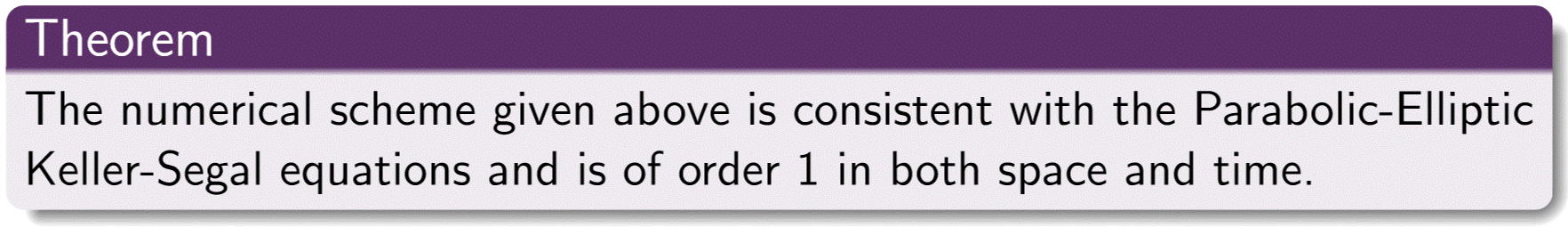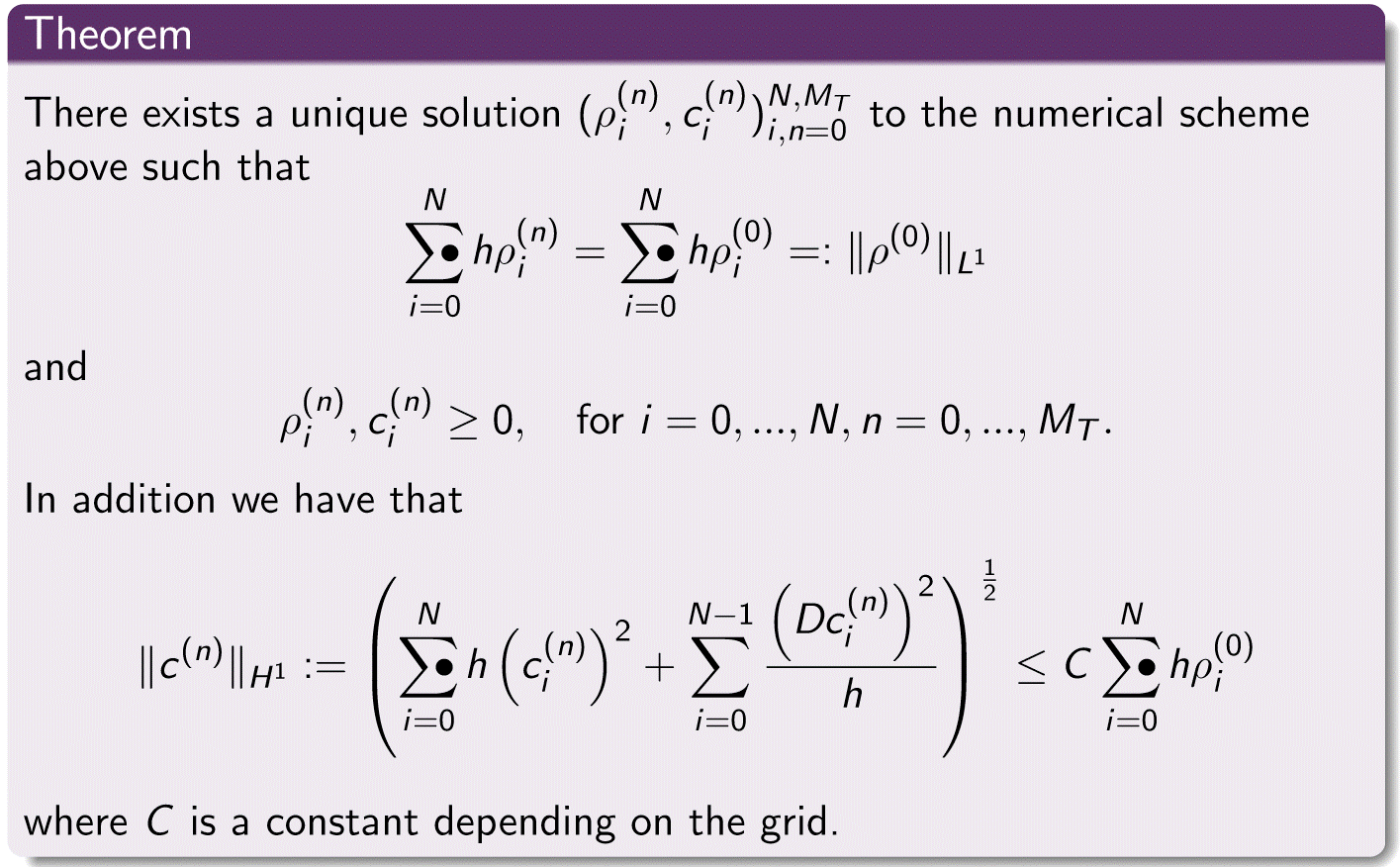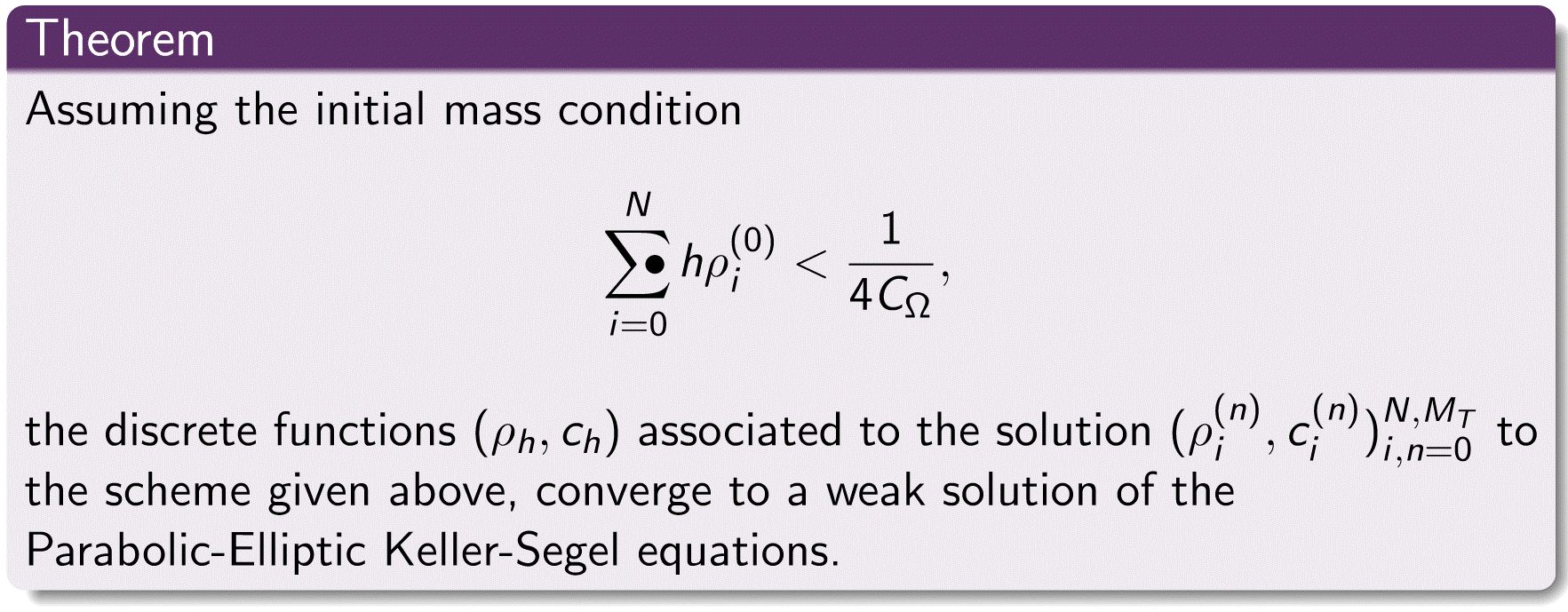# Numerical Analysis

#### Numerical Scheme

The numerical scheme for which we have proven the analytical results below, and which we used for our numerical simulations is given by$\frac{\rho_i^{(n+1)} -\rho_i^{(n)}}{\Delta t} = \frac{1}{h^2}\left(D^2\rho_i^{(n+1)}-\rho_i^{(n+1)}D^2c_i^{(n+1)}-\left(Dc_{i-1}^{(n+1)}\right)^+D\rho_{i-1}^{(n+1)}+\left(Dc_{i}^{(n+1)}\right)^-D\rho_{i}^{(n+1)}\right)$

and$\frac{D^2c^{(n+1)}_{i}}{h^2}+\rho_i^{(n+1)}-c_i^{(n+1)}=0$

where$D^2u_i=u_{i+1}-2u_i+u_{i-1},\qquad Du_i=u_{i+1}-u_i$

We proved that such a scheme is consistent with the Parabolic-Elliptic Keller-Segel equations. The statement of the theorem is as follows#### Convergence

We proved two major results for the numerical scheme given above which together give convergence of the scheme to a weak solution of the Keller-Segel equations. The first involves proving that the scheme has a unique solution at each time step. For this we proved the following theorem, which also gives us conservation of mass, positivity of the solution and a uniform bound on the discrete$H^1$ norm of the approximation to the chemical concentration$c$. The proof of existence involves a linearisation of the scheme together with an application of the Brouwer fixed point theorem and was adapted from the proof given by Filbet in the finite volume setting . In the following we have made use of the trapezium rule, so for notational convenience we define the ''trapezium sum" as follows$\sum_{i=0}^{N}\mathop{}{\mkern-23mu \bullet}\: a_i = \frac{a_0+a_N}{2} + \sum_{i=1}^{N-1} a_i.$

The statement of the first theorem isThe second result was split into two parts, the first of which was to prove convergence in some sense of the unique solution to the scheme to a couple$(\rho,c)$ as the time step$\Delta t$ and grid size$h$ approach zero, and finally we proved that this$(\rho,c)$ is a weak solution to the Keller-Segel equations. For this we first defined discrete functions$\rho_h$ and$c_h$ associated to the solution of the scheme as piecewise constant functions taking corresponding values at grid points and discrete time steps. We also define piecewise constant approximations$d\rho_h$ and$dc_h$ to the derivatives with$d\rho_h(t,x) =\frac{\rho_{i+1}^{(n)}-\rho_i^{(n)}}{h}$ on$(t^n,t^n]\times[x_i,x_{i+1})$. We then needed some a-priori estimates on discrete norms of$\rho_h$ and$c_h$ and due to the form of the non-linearity in the parabolic equation, in order to pass to the limit we required strong convergence of$\rho_h$ to$\rho$ but only weak convergence of$c_h$ to$c$ and of the approximations to the derivatives,$d\rho_h$ and$dc_h$ to$\rho_x$ and$c_x$. This result can be summarised in the following theorem, the proof of which was mostly adapted from .#### References

 F. Filbet, A finite volume scheme for the Patlak-Keller-Segel chemotaxis model, (2005)